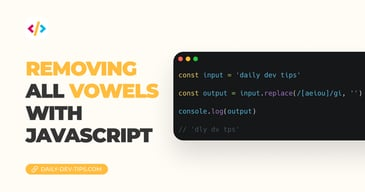Subscribe

# JavaScript basics comparison operators

••••••• + 159 devs liked it

✍️

Learning about comparison operators in JavaScript

25 Aug, 2021 · 1 min read

In this article on JavaScript basics, we'll look at comparison operators. These operators can be used to compare two values returning a boolean (true or false).

These are super handy for decision-making. Let's see which ones we can use:

OperatorComparisonExample
`==`Equal to`8==8 // true`
`5==8 // false`
`'5'==5 // true`
`'f'=='f' // true`
`!=`Not equal to`8!=8 // false`
`5!=8 // true`
`'5'!=5 // false`
`'f'!='f' // false`
`===`Strict equal to`8===8 // true`
`'5'===5 // false`
`'f'==='f' // true`
`!==`Strict not equal to`8!==8 // false`
`'5'!==5 // true`
`'f'!=='f' // false`
`>`Greater than`5>8 // false`
`8>5 // true`
`5>5 // false`
`<`Less than`5<8 // true`
`8<5 // false`
`5<5 // false`
`>=`Greater than or equal to`5>=8 // false`
`8>=5 // true`
`5>=5 // true`
`<=`Less than or equal to`5<=8 // true`
`8<=5 // false`
`5<=5 // true`

## JavaScript equal to operator permalink

This operator is used to evaluate two values. However, they don't have to be of the same type. Meaning we can assess if a string is equal to a number!

```````5` == 5; // true
5 == 5; // true``````

But it can also compare strings, for instance:

``````'string' == 'string'; // true
'String' == 'string'; // false``````

Note: It's capital sensitive, as you can see above!

## JavaScript not equal to operator permalink

Following this is the not equal to operator, which can evaluate if a comparison is not correct.

``````5 != 5; // false
8 != 5; // true
'8' != 5; // true
'String' != 'string'; // true
'string' != 'string'; // false``````

Then we have these two as strict versions, which should be preferred over the top ones. What this means is that it will check against the type as well.

``````5 === 5; // true
'5' === 5; // false``````

And the same works for the not equal to strict comparison.

``````5 !== 5; // false
8 !== 5; // true
'8' !== 5; // true``````

## JavaScript Greater and Less then permalink

Then we have the greater than and less than operators. These can be used to assess if a value is greater or less than the compared one.

Generally, these should only be used with number values.

``````8 > 5; // true
8 < 5; // false
5 > 8; // false
5 < 8; // true
5 > 5; // false``````

## JavaScript greater/less or equal to permalink

We can also use the above two comparisons to check whether something hits a threshold.

We want to evaluate if a value is greater than or equal to a certain number?

``````5 >= 5; // true
8 >= 5; // true``````

Meaning our number is greater than or equal to 5, which is the case in the above example.

This can also be used for checking less than operations.

``````5 <= 5; // true
3 <= 5; // true``````

Tweet this tip
••••••• + 159 devs liked it

### Removing all vowels with JavaScript

3 Dec, 2022 · 2 min read### 10 games to learn JavaScript

2 Dec, 2022 · 3 min readJoin 2101 devs and subscribe to my newsletter

• 1000 articles written
• 2101 devs subscribed
• 529116 words written